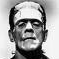# QlikView App Dev

Discussion Board for collaboration related to QlikView App Development.

Announcements
Support Case Portal will move to Qlik Community Oct 4. Temporary case outage Oct 3. READ DETAILS
cancel
Showing results for
Did you mean:Contributor II

## Invalid Dimension error ?

I am fairly new and not sure why I get error Invalid Dimension for this expression in my table when I have others similar working.

=Sum({<Group={'CG'},TS = {'QS'},PCat = {'Premium'}>}Revenue) + Sum({<Group={'CG'},brand = {'Services'}>}Revenue)+ Sum({<Group={'CG'},brand = {'Wks'}>}Revenue) + Sum({<Group={'CG'},brand = {'Options'}>}Revenue) / Sum({<Group={'CG'}>}Revenue

If there is a better way to write this as well I am eager to learn.

Labels (1)
• ### Sum divide invalid dimension

2 Solutions

Accepted SolutionsMVP
Are you using that as a measure or as a dimension? If you try to use it as a dimension I understand why you get an error. An expression can only return one result. A simple sum(something) cannot be used as a dimension. You need to specify one or more fields that need to be used to calculated the sums over. For that you need to use the Aggr function: Aggr(sum(Revenue), MyDimension1, MyDimension2, ... etc).

talk is cheap, supply exceeds demandMVP
Great. Glad you got it sorted. Could you mark a post as the solution? That makes it easier for other users to find solutions to their questions.

talk is cheap, supply exceeds demand
7 RepliesMaster II

Since you are adding four expressions, I would try each individual expression to see which one causes the error.

If that fails, you might try using rangesum instead of +.

Now i see the division.

Do you really mean to do:

Sum({<Group={'CG'},brand = {'Options'}>}Revenue) / Sum({<Group={'CG'}>}Revenue

Or did you want the four expressions summed first and then divide?MVP
There was a missing closing parenthesis at the end of the expression:

=Sum({<Group={'CG'},TS = {'QS'},PCat = {'Premium'}>}Revenue) + Sum({<Group={'CG'},brand = {'Services'}>}Revenue)+ Sum({<Group={'CG'},brand = {'Wks'}>}Revenue) + Sum({<Group={'CG'},brand = {'Options'}>}Revenue) / Sum({<Group={'CG'}>}Revenue )

talk is cheap, supply exceeds demandContributor II
Author

So I think maybe the expression may not be the issue as I tried just Sum({<Group={'CG'}>}Revenue) and still get the error, even though I have this expression in another column where it works without error.  If I change the expression to just =Revenue the error is gone.Contributor II
Author
Sorry that was in my real expression just didnt paste over.MVP
Are you using that as a measure or as a dimension? If you try to use it as a dimension I understand why you get an error. An expression can only return one result. A simple sum(something) cannot be used as a dimension. You need to specify one or more fields that need to be used to calculated the sums over. For that you need to use the Aggr function: Aggr(sum(Revenue), MyDimension1, MyDimension2, ... etc).

talk is cheap, supply exceeds demandContributor II
Author

Thanks so much, built it as a measure and it works.MVP
Great. Glad you got it sorted. Could you mark a post as the solution? That makes it easier for other users to find solutions to their questions.

talk is cheap, supply exceeds demandCommunity Browser